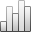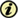Acta Stereologica

0351-580XMore Statisticssince 05 December 2013 :
View(s): 195 (4 ULiège)
Jiří Janáček

### Errors of spatial grids estimators of volume and surface area

(Volume 18 (1999) — Number 3 - Nov. 1999)
Article#### Abstract

Errors of the volume and surface area estimators that use IUR spatial grids of points, lines and planes are calculated using geometric characteristics of the objects under study. Coefficients in trend terms of asymptotic formulae for variances of volume estimators that use simple orthogonal grids are calculated. The variance of surface area estimation using spatial grids of lines has two components, the variance due to orientation and the residual variance. Upper bounds of the component of the error of surface area estimator due to the orientation are calculated for various sets of directions of grid lines. Coefficients in trend terms of asymptotic formulae for residual variances of the surface area estimators using triple orthogonal spatial grids of lines are calculated.

Keywords : spatial grid, surface area, systematic sampling, variance, volume